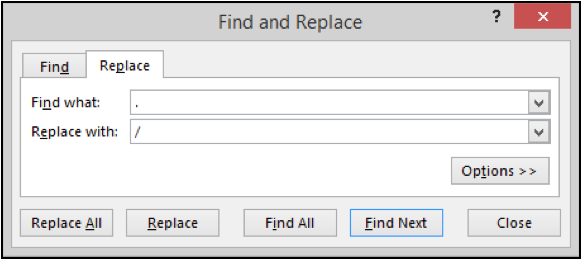# How to delete/remove a word in ExcelIn this article, we will learn How to delete / remove a word in Excel.

Scenario:

In Excel, removing or replacing a particular word is very easy. For example removing .com from all the cells in the current sheet. Or replacing a word with another word within excel. For these problems we use the Find and Replace option in excel.

Find and Replace all in Excel

Find and Replace options are common functions in excel. And these are the common practices to use these options in Excel. Let's get this one by one.

You can locate the find option using two ways

1. Go to Home tab > Find & Select > Find
2. Use Ctrl + F shortcut to find dialog box.

You can find the replace option in excel from the below mentioned ways.

1. Go to Home tab > Find & Select > Replace
2. Use Ctrl + H shortcut to replace dialog box.

Example :

All of these might be confusing to understand. Let's understand how to use the function using an example. Here we will learn how to access the find and replace dialog box. These two options are very similar to use in Excel.

First we learn how to access the Replace option. Go to Home tab > Find & Select > Replace or use Ctrl + HFill the value to replace and the value to replace with. If you wish to delete / remove the word, then leave the replace with option blank.

Here are all the observational notes using the formula in Excel
Notes :

1. You can replace one by one or all at once in excel.
2. Find options only locates the occurrence of words in the sheet.

Hope this article about How to delete / remove a word in Excel is explanatory. Find more articles on calculating values and related Excel formulas here. If you liked our blogs, share it with your friends on Facebook. And also you can follow us on Twitter and Facebook. We would love to hear from you, do let us know how we can improve, complement or innovate our work and make it better for you. Write to us at info@exceltip.com.

Related Articles :

Find the partial match number from data in Excel : find the substring matching cell values using the formula in Excel

How to Highlight cells that contain specific text in Excel : Highlight cells based on the formula to find the specific text value within the cell in Excel.

Conditional formatting based on another cell value in Excel : format cells in Excel based on the condition of another cell using some criteria.

IF function and Conditional formatting in Excel : How to use IF condition in conditional formatting with formula in excel.

Excel REPLACE vs SUBSTITUTE function: The REPLACE and SUBSTITUTE functions are the most misunderstood functions. To find and replace a given text we use the SUBSTITUTE function. Where REPLACE is used to replace a number of characters in string…

Replace text from end of a string starting from variable position : To replace text from the end of the string, we use the REPLACE function. The REPLACE function use the position of text in the string to replace.

Popular Articles :